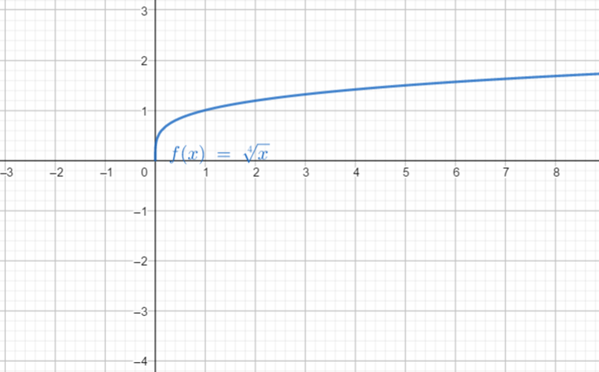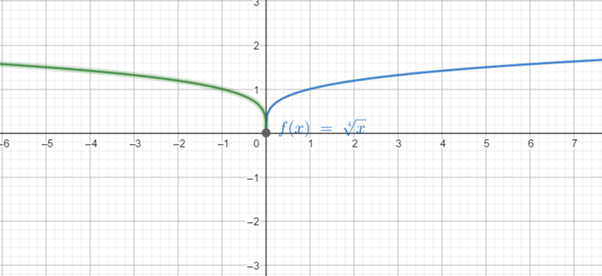# To graph: the function f ( x ) = − x 4 using the graph of a standard function and then applying transformations.### Precalculus: Mathematics for Calcu...

6th Edition
Stewart + 5 others
Publisher: Cengage Learning
ISBN: 9780840068071### Precalculus: Mathematics for Calcu...

6th Edition
Stewart + 5 others
Publisher: Cengage Learning
ISBN: 9780840068071

#### Solutions

Chapter 2.5, Problem 31E
To determine

## To graph: the function f(x)=−x4 using the graph of a standard function and then applying transformations.

Expert Solution

### Explanation of Solution

Given information:

the function is f(x)=x4

Graph:

To plot the graph of f(x)=x4 ,use the plotting points method, substitute different value of x on f(x)=x4 to determine corresponding value of f(x) .

The table is thus obtained as:

 x 16 81 256 1 y 2 3 4 1

now plot each point of x along the horizontal axis and the corresponding value of f(x)=y along vertical axis and connect each point to form a smooth curve.

The graph of f(x)=x4 is obtained as:Now, the graph of f(x)=x4 is the graph of f(x)=x4 reflectedin y -axis.

The graphof f(x)=x4 is obtained as:Interpretation:

From the above graph it can be obtained that the graph of f(x)=x4 is obtained by reflectingthe graph of f(x)=x4 in y -axis.

### Have a homework question?

Subscribe to bartleby learn! Ask subject matter experts 30 homework questions each month. Plus, you’ll have access to millions of step-by-step textbook answers!